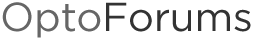# How will a for loop react if I change the value of the index out of the initial loop range within the loop?

If I take a simple for loop
for Index = 36 to 45 step 1
and alter the index within the loop
if (index == 45) then index = 146
how will the loop respond? Will it finish the current iteration and then exit (ideal behavior)? Exit immediately? Crash the chart?

The reason I’m asking is that I’m adding an additional device to a system, where the current related inputs are using consecutive indexes on several related arrays to keep track of relevant values. Now I need to add an additional device, but the next unused index is 146 while the existing devices in the same area are 36 through 44, so the easiest way to modify the code would be to change the for loops to 36 to 45, and add an if statement at the start of the loop to bump the index to 146 if it equals 45. (Well, unless there’s a way to have two ranges in one for statement (for index = 36 to 44, 146))

We do something like this to set two (or more) ranges within an index.

for nIndex = 100 to 150 step 1
if ((IsWithinLimits(nIndex, 100, 104)) or //nIndex 105 is should be ignored
(IsWithinLimits(nIndex, 106, 150))) then
//Code for whatever needs to happen here
endif
next

Hope that helps.

1 Like